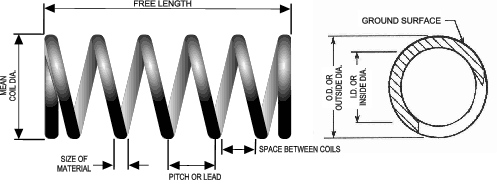# Design Considerations for Compression Springs

A Compression Spring is an open-coil helical spring that offers resistance to a compressive force applied axially. The illustration and formula below show the various design parameters required to select the appropriate spring for your application:## Measured Spring Rate = lbs. of force / inches of deflection. (lbs./in.)

Spring can be measured by taking the difference in force at 80% maximum deflection and 20% maximum deflection and dividing by the difference in deflection. The spring rate is constant over the central 60 percent of the deflection range. Because of end-coil effects, the first 20% of deflection range has a considerably lower spring rate. The final 20% of deflection shows considerably higher spring rate. If possible, critical loads and rates should be specified within the central 60% deflection range.

## Calculated Spring Rate (R) = Gd4/8nD3 pounds per inch where:

R = Rate, pounds of load per inch of deflection
G = modulus of rigidity of material, pounds per square inch
d = wire diameter, inches
n = number of active coils, which is two less than the total number of coils
D = mean coil diameter, inches

## Maximum Deflection = maximum load / spring rate

The maximum load value (or alternatively the design load) will be provided to you in the spring selection database.